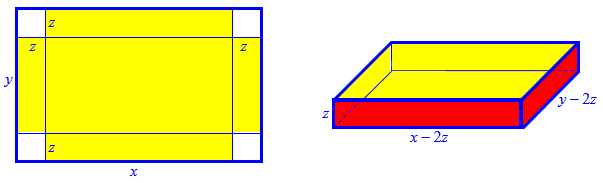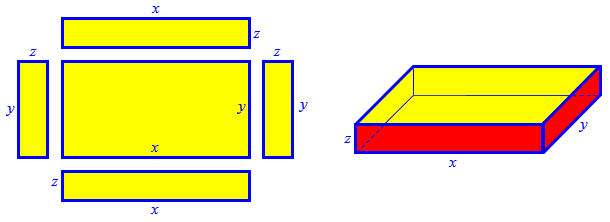# Maximum Volume of a Box: Two Interpretations

#### (A new question of the week)

Often the hardest part of solving a problem is interpreting what it means. Math is precise; human language can be ambiguous, and assumptions can be hidden. Today, we look at a multi-variable calculus problem that looked enough like a classic single-variable maximization problem to fool the reader into not taking it at face value – but as a result, we get to talk about two problems at once!

## First interpretation

Here is the question, which came from Stefan last month:

Find the maximum volume of a box with its top removed which is supposed to be made out of 12 m2 of cardboard.

Now I’m not sure what exactly to do.

Should I write the function for volume and then find the extreme values?

12 = x*y, x = 12/y

V = (y – 2z)(x – 2z)z

V = (12/y – 2z)(y – 2z)z

Doing this and finding partial derivatives ∂V/∂x and ∂V/∂y I get x, y = √12 and z1 = 0.57 and z2 = 1.73

V1 = 3 and V2 = 2.91042169×10-5, I know that both of these aren’t correct.

How do you know that both these solutions are incorrect? If an answer was provided, what was it?

The problem is not entirely clear. You seem to be reading it this way: “Find the maximum volume of a lidless box made by starting with a rectangle of area 12 m2, cutting squares out of the corners and folding up to make the sides.” This is a fairly common type of problem, but is not the only way the words of the problem could be interpreted.

Solving the problem as you interpreted it, I get the same results you get, though when I find the exact answers (not just using a calculator and rounding), my answers are a little more than 3 for the greater (which must be the maximum volume) and exactly 0 for the lesser.

Here is Stefan’s interpretation of the problem, showing the variables he has in mind:Let’s pause and do the work as Stefan presumably did it. He has found an expression for the volume V as a function of length x, width y, and height z; and he wants to maximize it. A problem is commonly given in this form with specific values for x and y, to be solved with only one variable. To find the maximum here, with two unknowns, we have to take the derivative of V with respect to one variable at a time, and set both to zero. If you are not familiar with this, a special symbol is used in place of the d‘s in the derivative, now called a partial derivative, just to remind us that other variables are being treated as constants. Here are the function and its two derivatives:

$$V = \left(\frac{12}{y} – 2z\right)(y – 2z)z = 4z^3-2yz^2-24y^{-1}z^2+12z$$

$$\frac{\partial V}{\partial z} = 12z^2-4yz-48y^{-1}z+12$$

$$\frac{\partial V}{\partial y} = -2z^2+24y^{-2}z^2$$

Setting these equal to zero, we have a system of equations, $$\left\{\begin{matrix}12z^2-4yz-48y^{-1}z+12=0\\ -2z^2+24y^{-2}z^2=0\end{matrix}\right.$$

To solve this, we can rewrite the second equation as $$z^2\left(\frac{24}{y^2}-2\right) = 0$$ so that $$z=0$$ or $$y=\sqrt{12}=2\sqrt{3}$$. In the former case, the first equation becomes $$12=0$$, so it is impossible (and the volume would be zero anyway). In the latter case, we find that $$x=\frac{12}{y}=\frac{12}{2\sqrt{3}}=2\sqrt{3}$$, and the first equation becomes $$12z^2-16\sqrt{3}z+12=0$$ which factors as $$4(3z-\sqrt{3})(z-\sqrt{3})=0$$

So the two solutions are $$x=2\sqrt{3},y=2\sqrt{3},z=\sqrt{3}\Rightarrow \\V = \left(\frac{12}{y} – 2z\right)(y – 2z)z = \left(\frac{12}{2\sqrt{3}} – 2\sqrt{3}\right)(2\sqrt{3} – 2\sqrt{3})\sqrt{3} = 0$$ and $$x=2\sqrt{3},y=2\sqrt{3},z=\frac{\sqrt{3}}{3}\Rightarrow \\V = \left(\frac{12}{y} – 2z\right)(y – 2z)z = \left(\frac{12}{2\sqrt{3}} – \frac{2\sqrt{3}}{3}\right)\left(2\sqrt{3} – \frac{2\sqrt{3}}{3}\right)\frac{\sqrt{3}}{3} = \frac{16}{3\sqrt{3}} = 3.0792$$

The dimensions of the box are $$x-2z=, y-2z=\frac{4\sqrt{3}}{3}, z=\sqrt{3}$$, so the ratio of dimensions is 4 : 4 : 3. But the numbers are such that, if the problem were intended to be taken this way, a different area than 12 probably would have been chosen!

## Second interpretation

Doctor Rick continued:

If this is not the intended answer, then we can try another interpretation. Perhaps the box is not made by starting with one rectangle of area 12 m2, but rather from five rectangles (four for the sides and one for the bottom) of total area 12 m2. (Alternatively you could start with three rectangles, one folded to make the bottom and a pair of opposite sides; it should come out the same.) I haven’t done the work for this yet.

In other words, if we take the problem literally, the box is made with 12 m2 of cardboard, with no scrap.

Stefan replied,

The solution is 4 m2, not cubed, so it might be an error in the book.

Is there a reason to use the other methods or can I always cut the corners?

Doctor Rick answered, having now done the work:

With the second interpretation of the problem, I get a maximum volume of 4 m3, so I’d say this is the intended meaning of the problem and only the units were a typo.

This isn’t about method — any valid method for solving a problem should give the same result — but about understanding the meaning of the problem. As I said, it is not worded very clearly. In the absence of clarity, I suppose you chose to interpret it as being like other problems you have seen, but the problem itself does not give us strong reason to take it that way.

Now that I am reasonably certain that I know what the problem is intended to be, would you like to try solving it? Again, this box is to be constructed from five rectangles of cardboard (for the front, back, left, right, and bottom of the box); the total area of the five rectangles is 12 m2. What is the maximum volume (or capacity) of the box, and what are the dimensions of this maximum-volume box?

Here is the reinterpreted problem; observe that the meaning of the variables has changed:Now we’ll have a considerably more complicated formula for the area that is 12 m2, but the volume formula is far simpler.

Stefan wrote back,

So the area would be xy + 2xz + 2yz = 12, x(y + 2z) = 12 – 2yz, x = (12 – 2yz)/(y + 2z)

V = xyz, V = (12yz – 2y2z2)/(y + 2z)

∂V/∂y = -2z2(y2 + 4yz – 12)/(y + 2z)2

∂V/∂z = -4y2(yz + z2 – 3)/(y + 2z)2

After solving these I get that y = ±2z and from this that z = ±1, z has to be positive, so I have y1 = 2 and y2 = -2,  and y also has to be positive, from this I get x = 2 and so V = 2*2*1 = 4

Is this how it’s meant to be done?

He again solved the area equation for x, and used that to write an expression for V as a function of y and z. The partial derivatives are correct. Setting them to zero, we can ignore the denominators, and the system we have to solve is $$\left\{\begin{matrix}y^2+4yz-12=0\\ z^2+yz-3=0\end{matrix}\right.$$

This looks hard to solve at first, but if we just subtract 4 times the second equation from the first, hoping to eliminate yz, everything goes right and we get $$y^2-4z^2=0$$ so that, indeed, $$y=\pm 2z$$. Just as Stefan said, we can ignore the negative case, plug $$y=2z$$ into the first equation to find that $$z=\pm 1$$. So he’s got it. We now have the right solution to the right problem. (The first interpretation was just good exercise.)

## Cleaning up

There was a three day lapse before Doctor Rick could reply:

I was writing back to you when the power went out at my house due to a storm. It came back on last night, so I can finally send you my reply:

I did something similar but not identical, so I can’t compare your work with mine line by line, but you did get the same results I got. (The results are “nicer” than the results following the other interpretation.)

I chose to solve for the height, z, rather than for x. I did this because the equations are symmetric under swapping of x for y, and this turned out to be helpful. My volume function was

V(x, y) = xy(12 – xy)/(2(x + y))

From ∂V/∂y = 0 I obtained the equation

y2 + 2xy – 12 = 0    (or y = 0)

Solving this directly isn’t so helpful, but I can immediately write the equation for ∂V/∂x by switching x and y:

x2 + 2xy – 12 = 0    (or x = 0)

Comparing the two equations, I see that x2 = y2, so y = ±x; and as in your work, I concluded that y = x because both must be positive. Putting y = x into the second equation, I got

3x2 – 12 = 0

so x = y = 2 (since x and y are non-negative). Plugging these into the function V(x, y), we get Vmax = 4; if x = 0 or y = 0, then V(x, y) = 0, clearly the minimum.

By the way, solutions to this sort of problem are typically “nice” in some sense. Here, the optimal dimensions of the box are $$1\times2\times2$$, and if we put two such boxes together we get a complete $$2\times2\times2$$ cube.

This site uses Akismet to reduce spam. Learn how your comment data is processed.